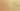MapToBooleanWithIf

Misconception:

An if statement is necessary for converting an expression such as a > b into a bool.Incorrect

To map a boolean expression to a bool, an if statement is necessary

Correct

To map a boolean expression to a bool, one can just use it

CorrectionHere is what's right.

In many cases, the condition used in an if statement is a boolean expression. In such cases, that expression already evaluates to a bool value. Taking that bool value and using an if statement to map from it to a bool value is entirely unnecessary. For example, this piece of code:

if x < 4:
is_small = True
else:
is_small = False

can be refactored into this:

is_small = x < 4

Note that Python allows non-booleans to be used as conditions in if-statements. For example, the following is an idiomatic way to check whether a list, lst in this snippet, is empty or not:

if lst:
non_empty = True
else:
non_empty = False

and is not equivalent to

non_empty = lst

OriginWhere could this misconception come from?

This misconception may stem from the student doing a case analysis:

• If this condition is True, what do we want to do?
• If the condition is False, what do we want to do?

SymptomsHow do you know your students might have this misconception?

In the following examples, assume that CONDITION is a boolean expression (e.g, is_happy(), i < len(lst), o is None, pacman.is_hungry(), or a and b for boolean a and b)

if CONDITION:
b = True
else:
b = False

if CONDITION:
return True
else:
return False

Python